NCERT Exemplar Class 8 Maths Solutions for Chapter 4 - Linear Equations In One Variable

NCERT Exemplar Class 8 Maths Solutions for Chapter 4 - PDF Download Free Download

NCERT Exemplar Class 8 Maths Chapter 4 Linear Equations in One Variable, is provided here for students to prepare for exams. These exemplars problems and solutions are designed by our experts in accordance with CBSE syllabus(2018-2019) for 8th standard students, which covers all the topics of Maths chapter 4.

The chapter Linear equations in one variable are one of the most important chapters for the students of class 8. In class 8 final examination one question of at least 5 marks are always asked from linear equations in one variable. To master the concepts of this chapter students are advised to solve the questions from NCERT exemplar.

Class 8 Maths NCERT Exemplar For Linear Equations in One Variable

Here in this chapter, students will solve exemplar problems based on following topics, like;

• Solving Equations having Linear Expressions on one Side and Numbers on the other Side
• Solve equations which have a variable on both sides
• Learn to reduce equations to a simpler form
• Learn to reduce equations to the Linear form
• Applications based on the equations

Solving exemplar for these topics will help students to score good marks in the main exams. Also, it is suggested to solve sample papers and previous year question papers which gives an idea of types of questions asked in the board exam from Linear Equations in One Variable. BYJU’S also provide exemplar books, notes, Maths NCERT solutions for 8th standard and question papers to help students practice well.

To get free PDF of NCERT exemplar, visit the below downloadable link.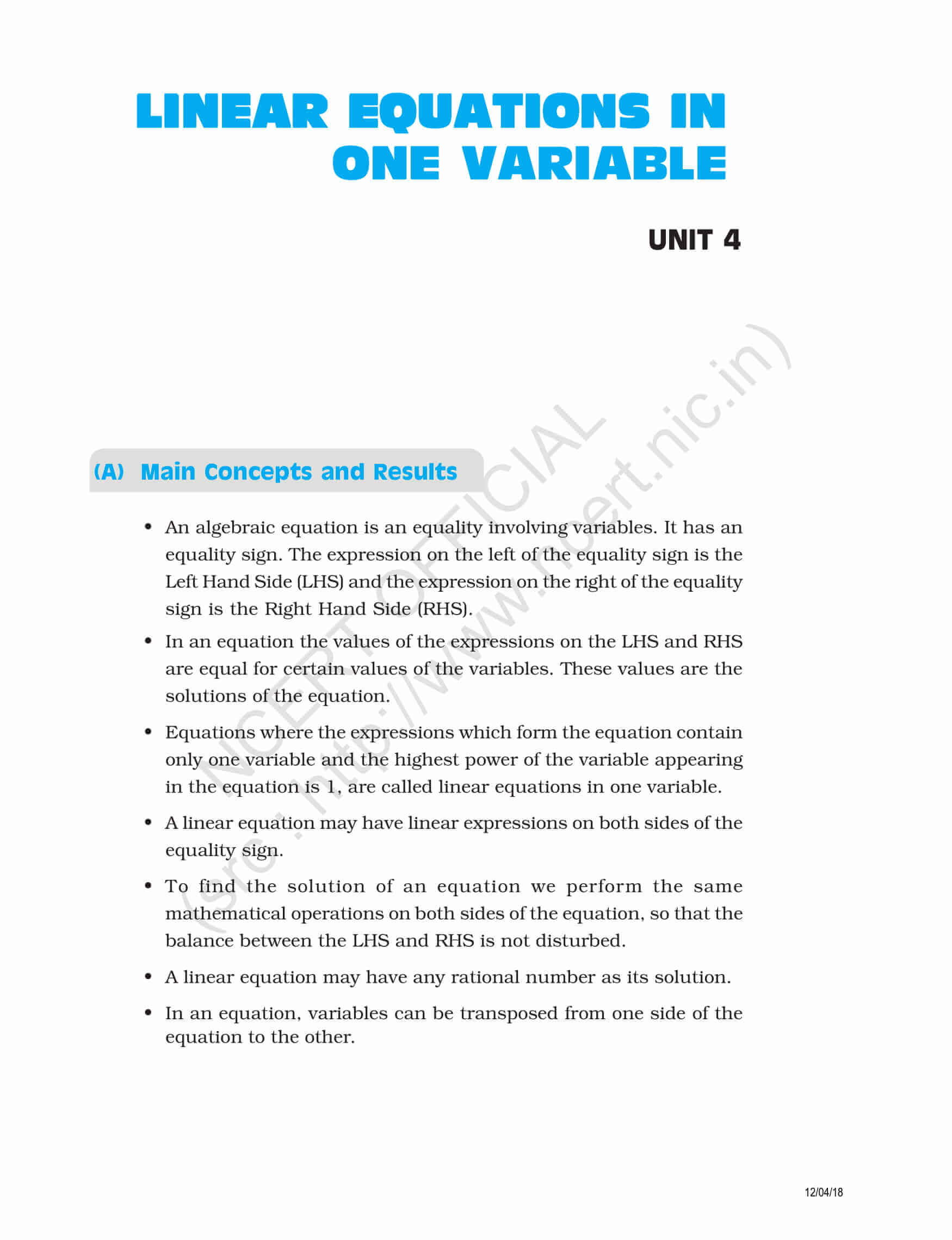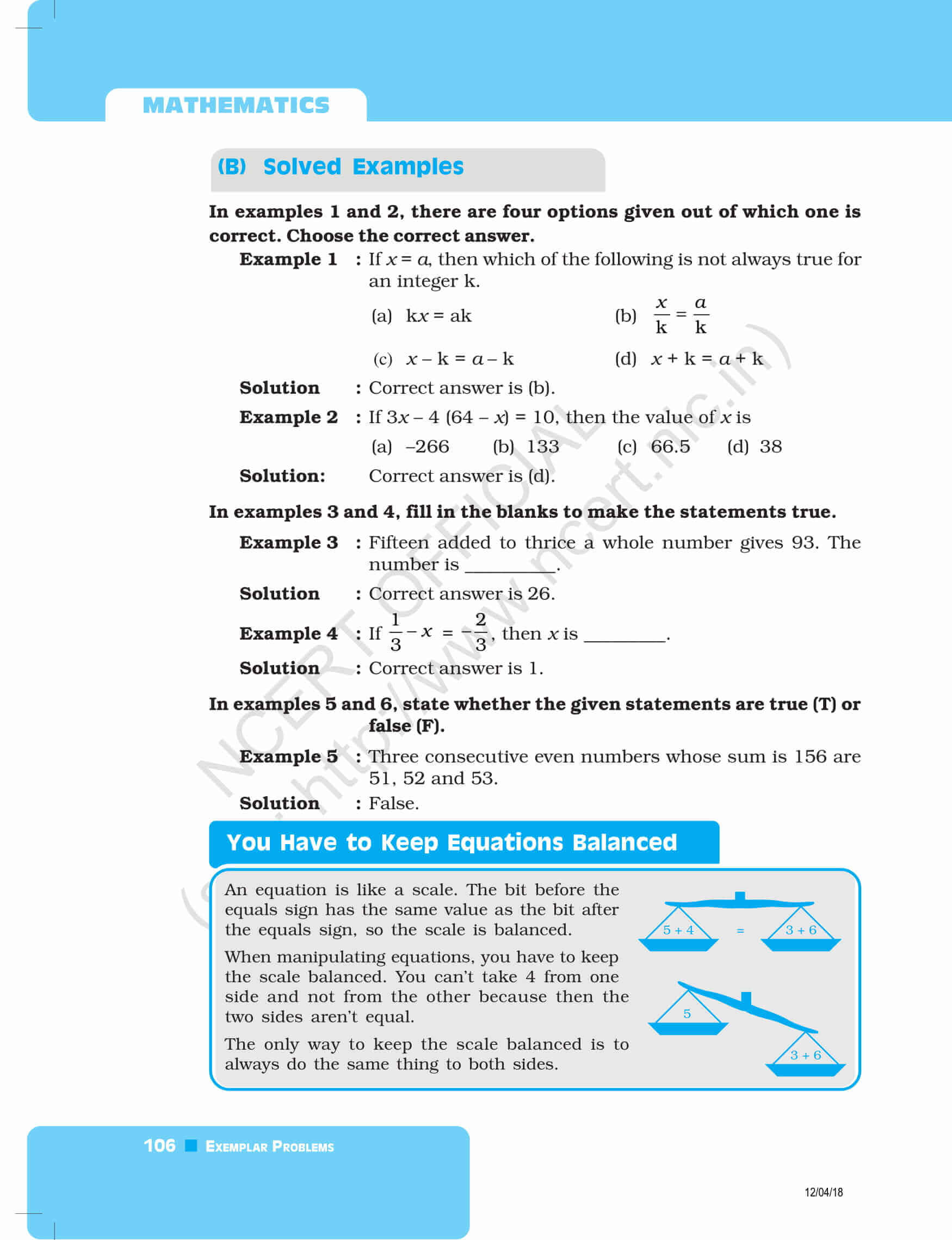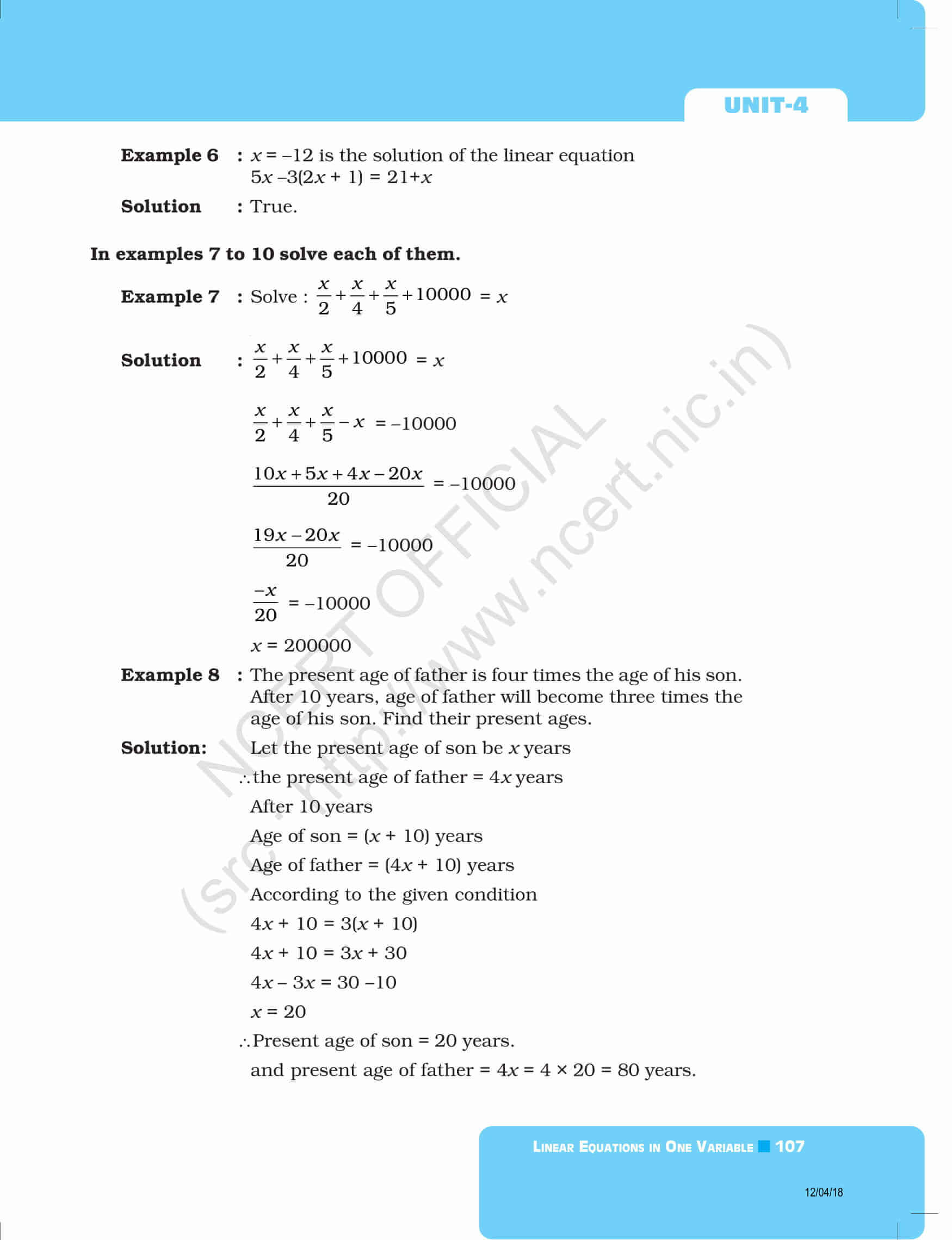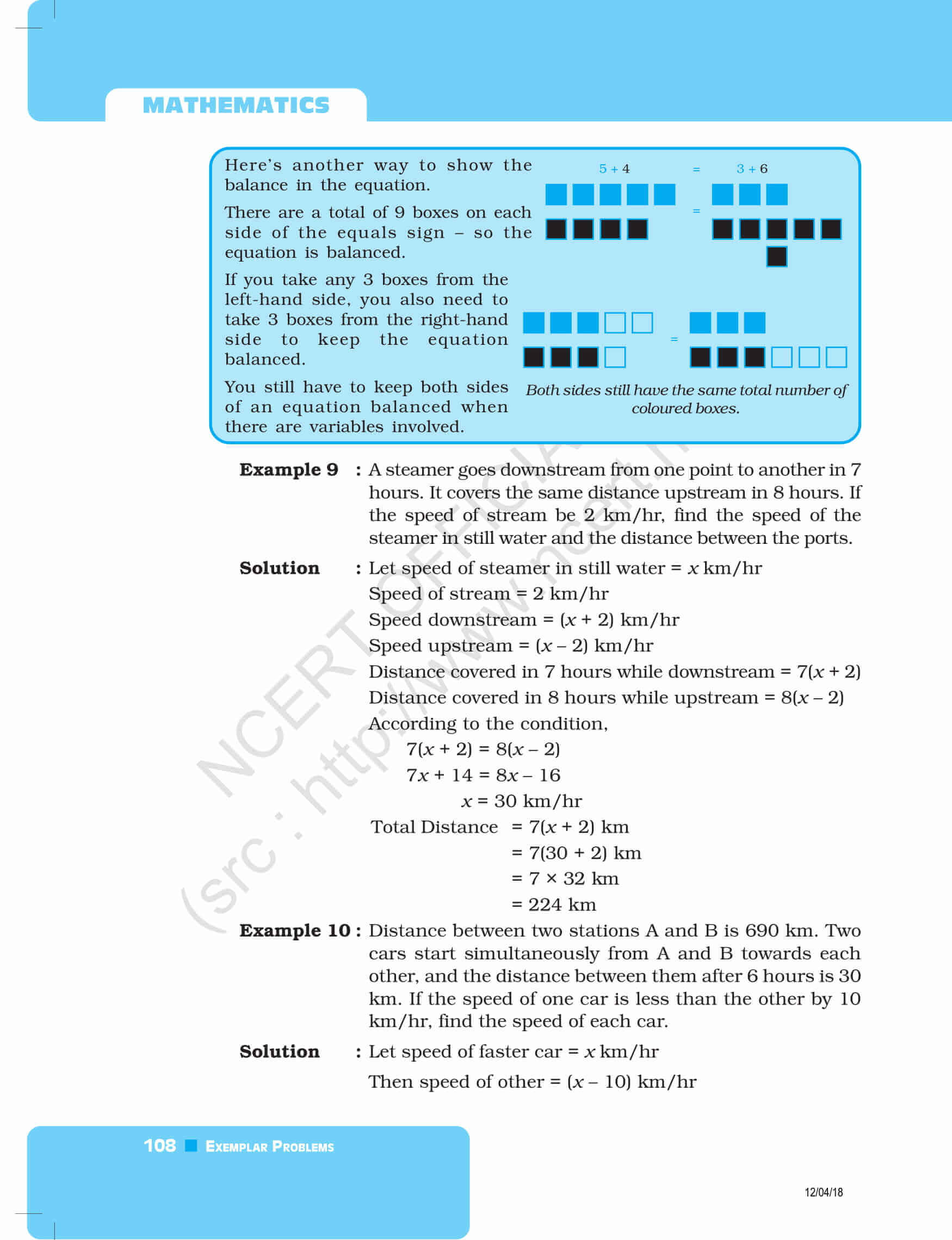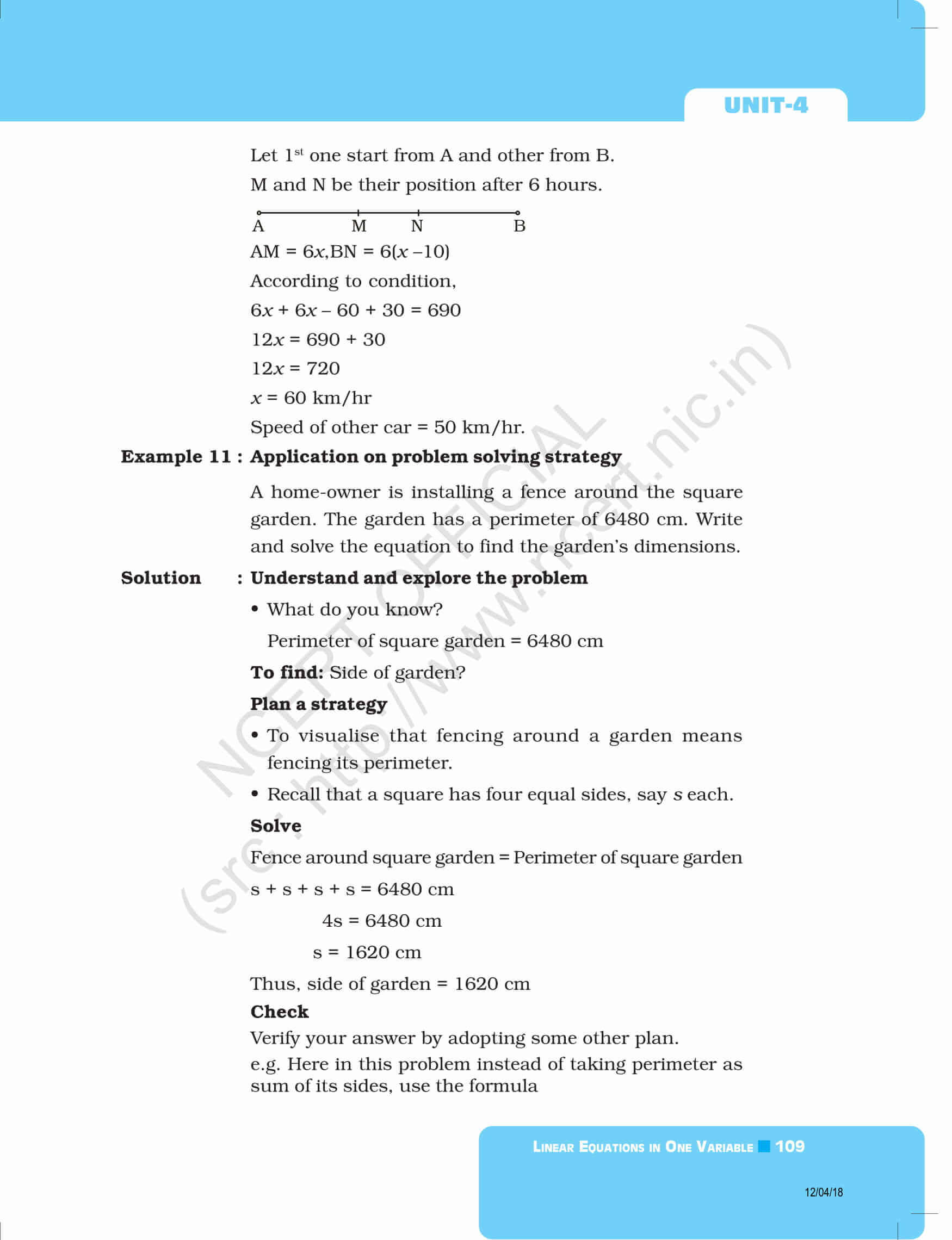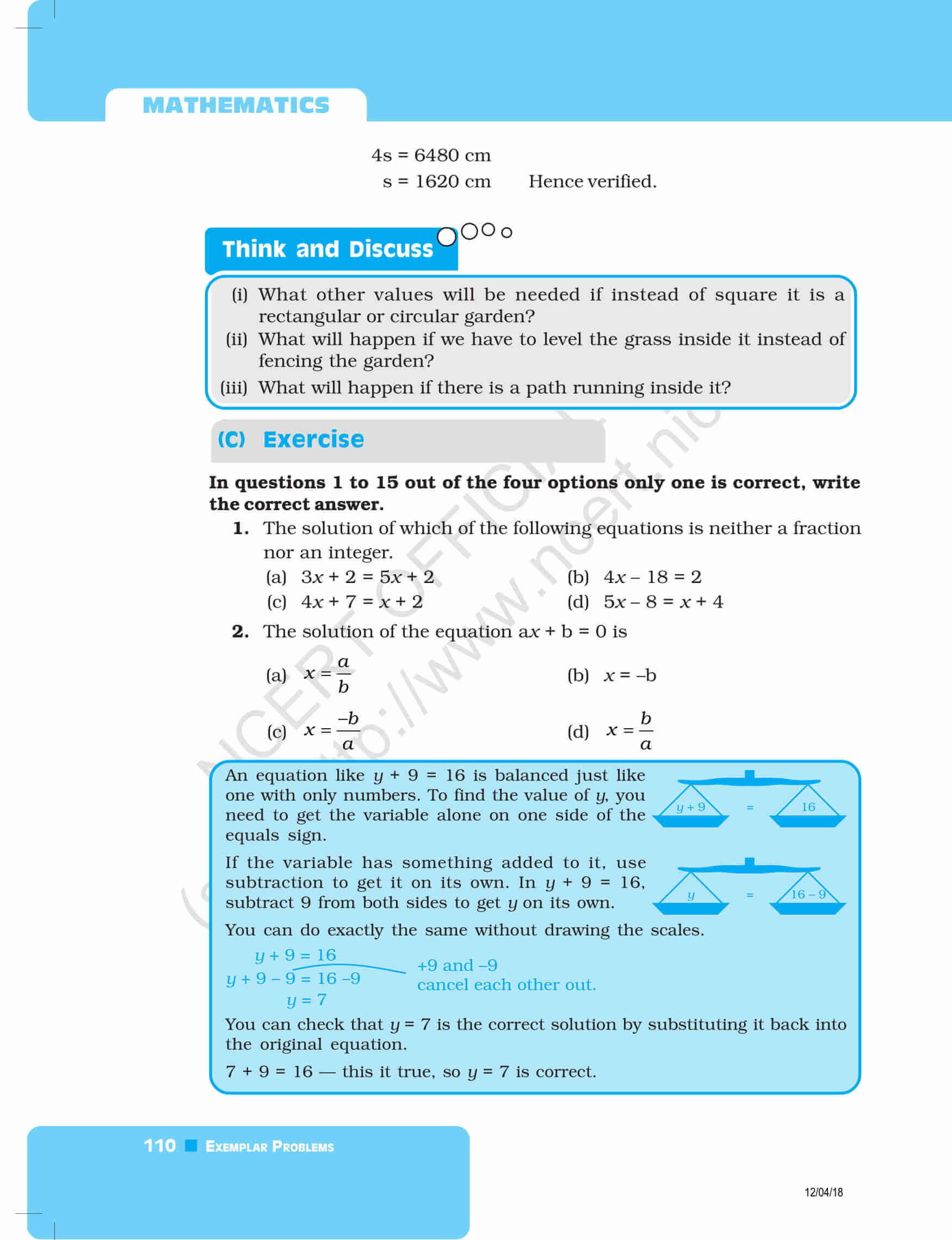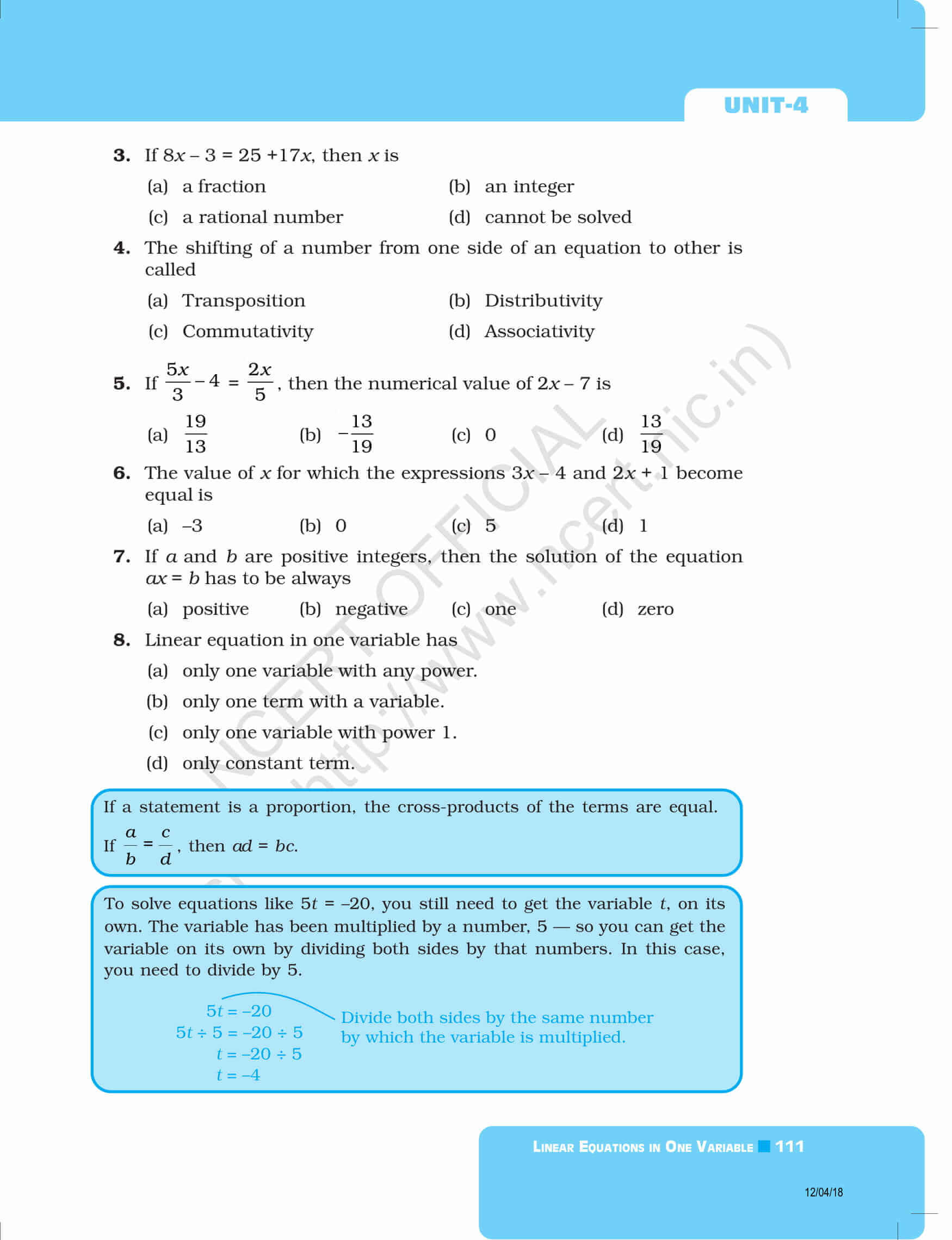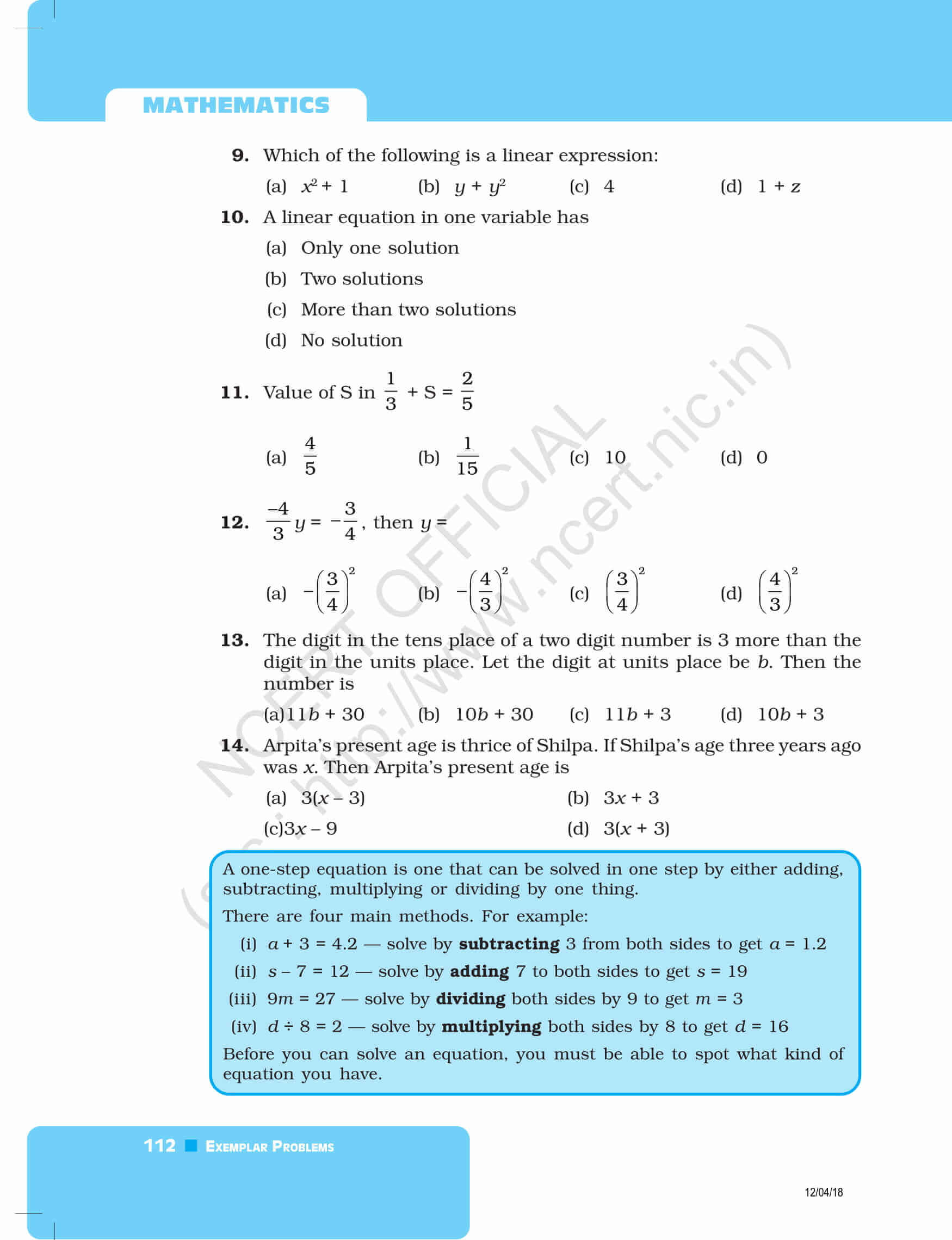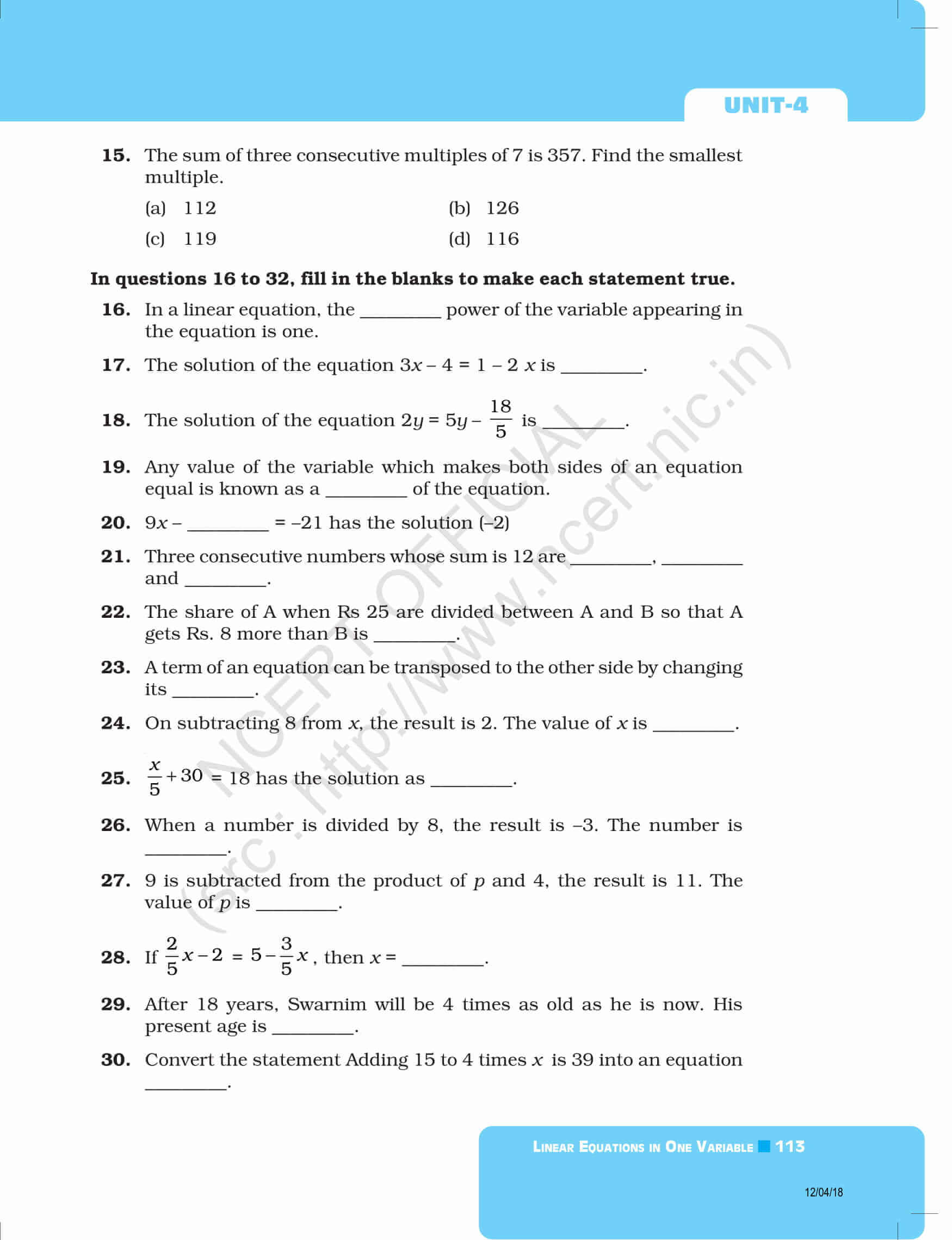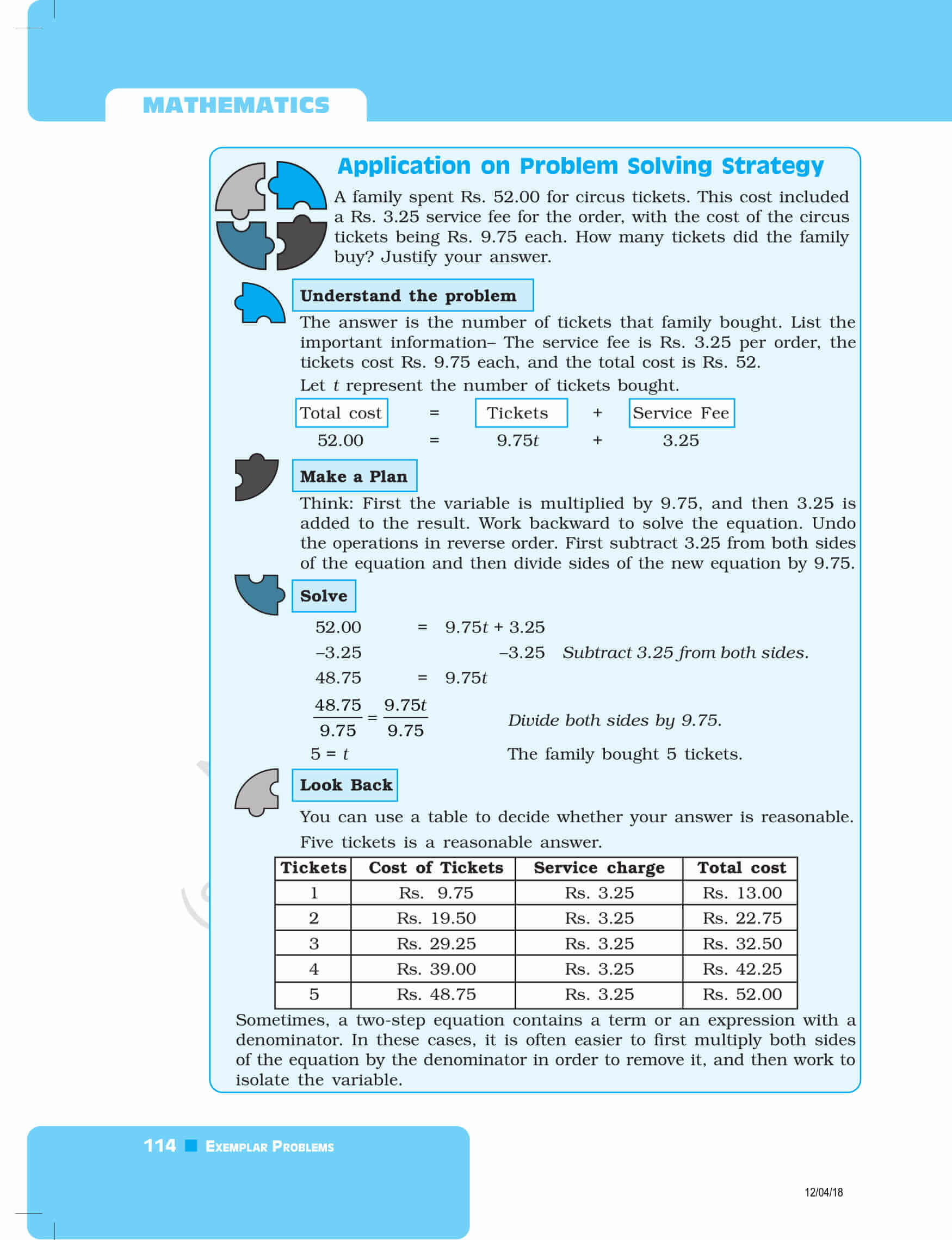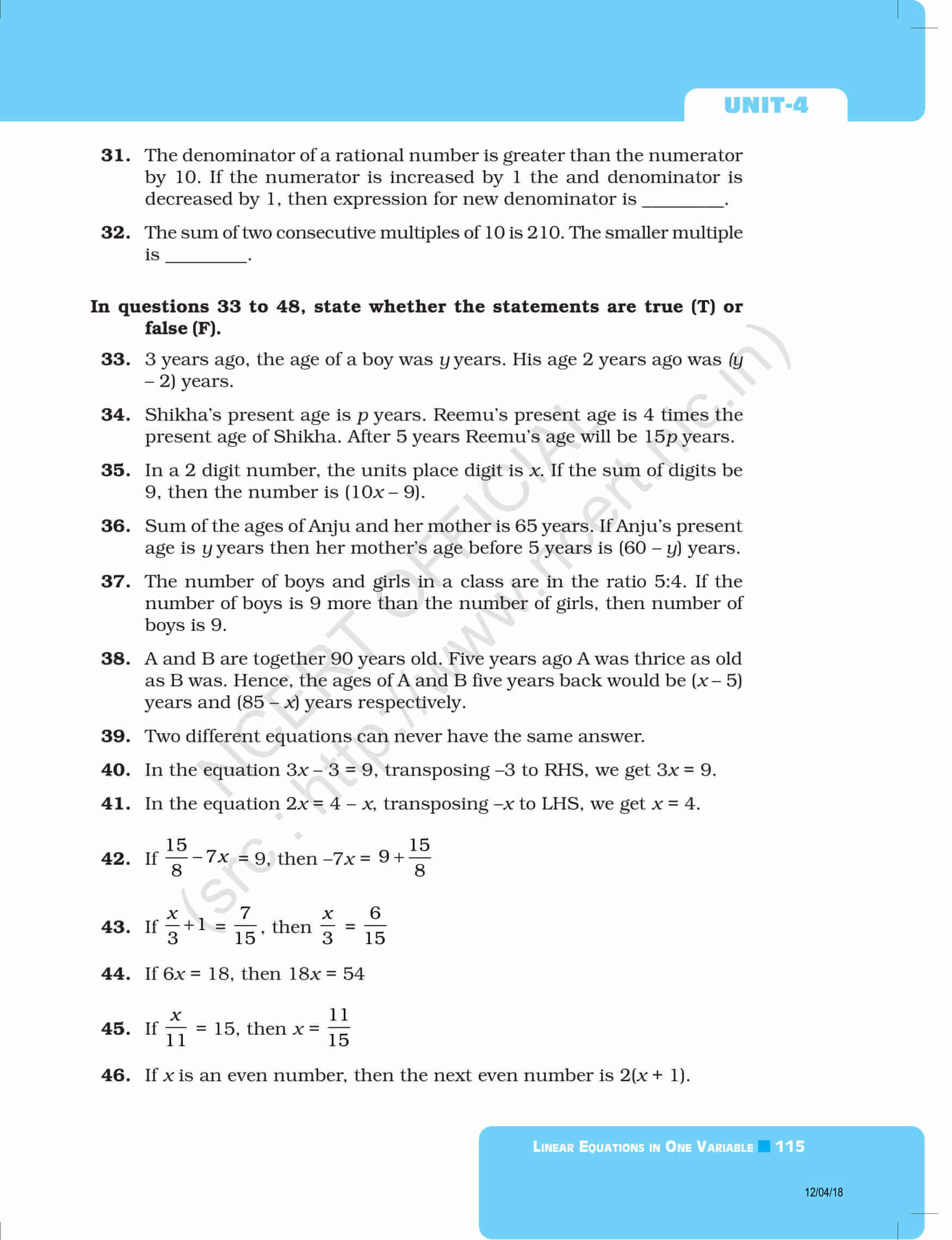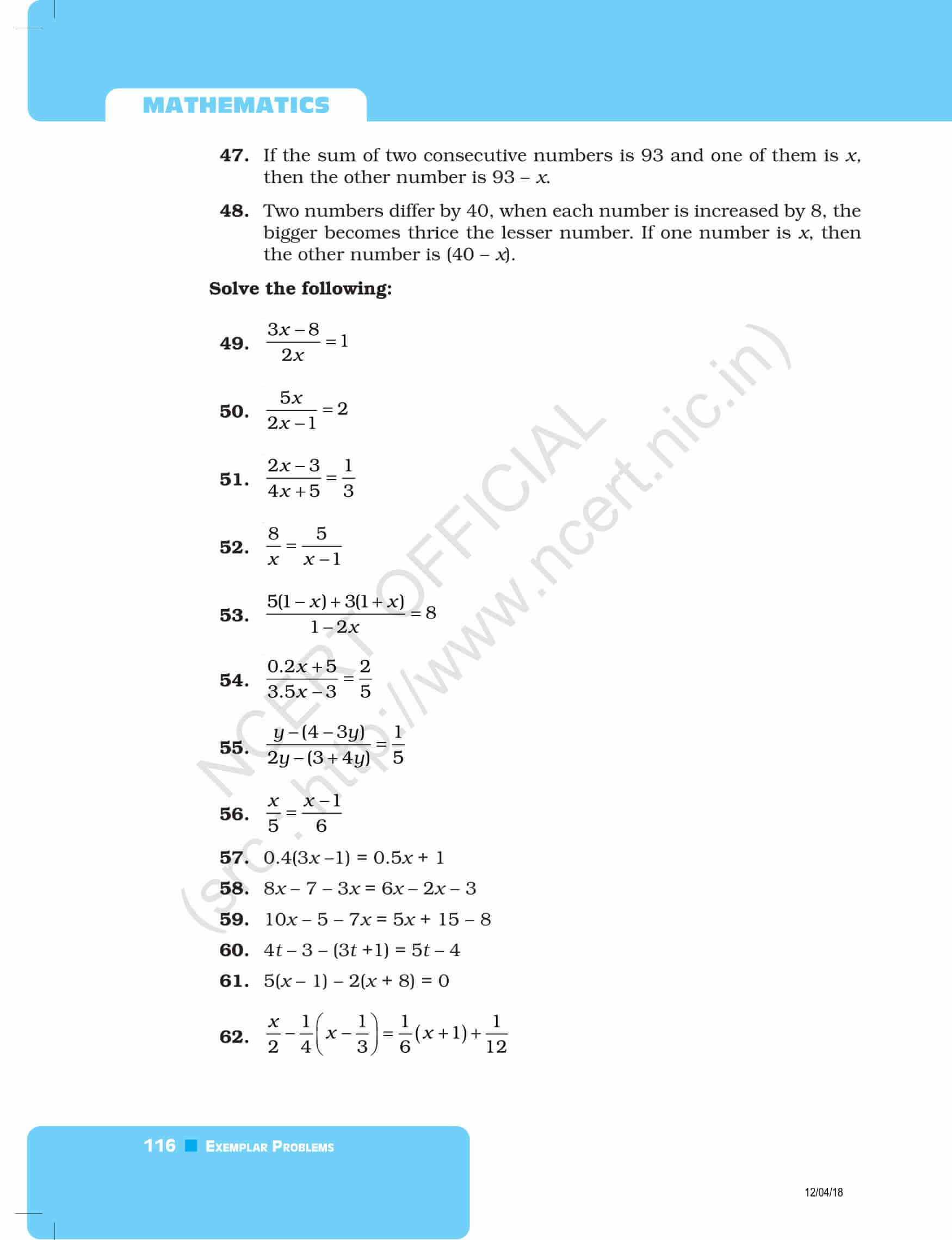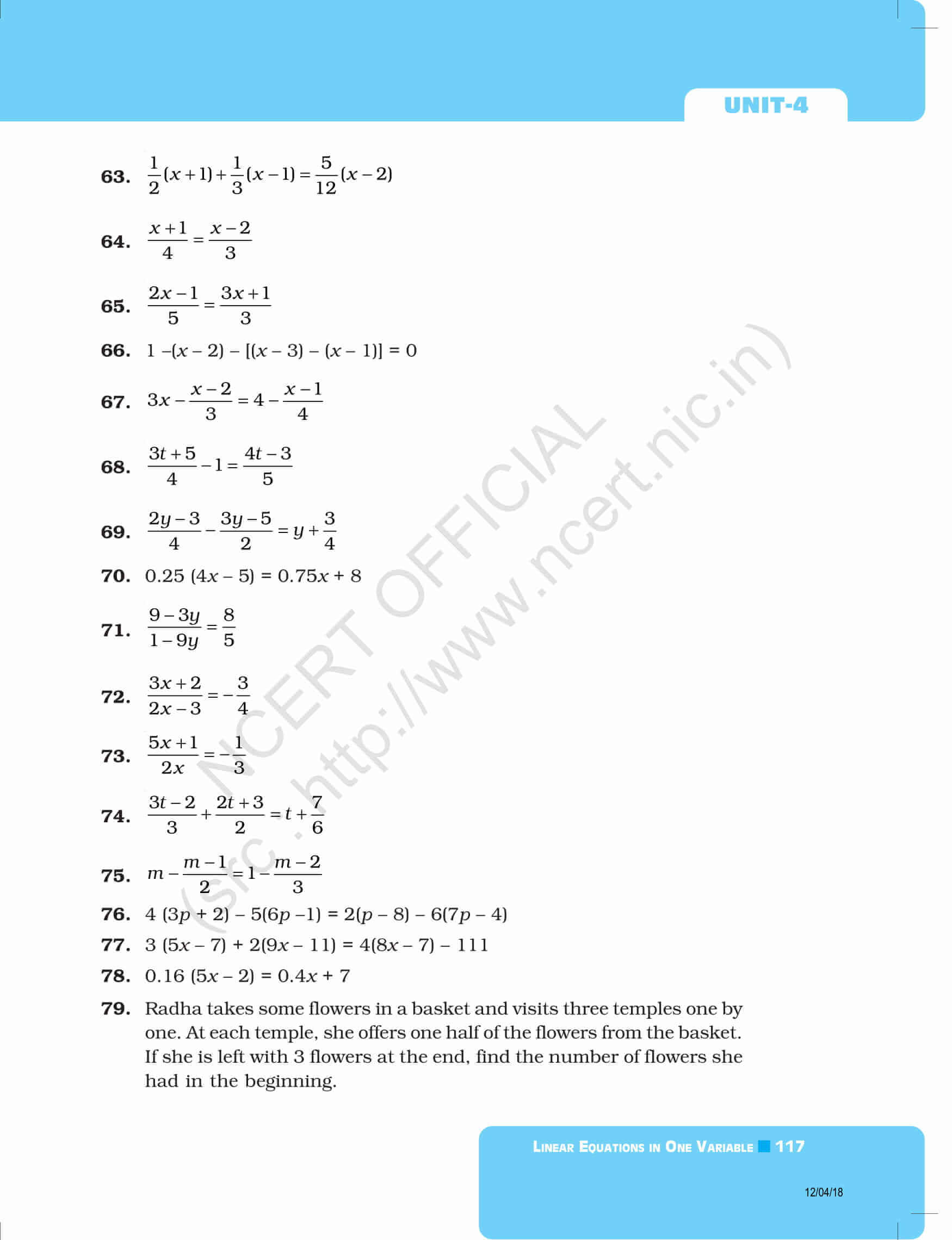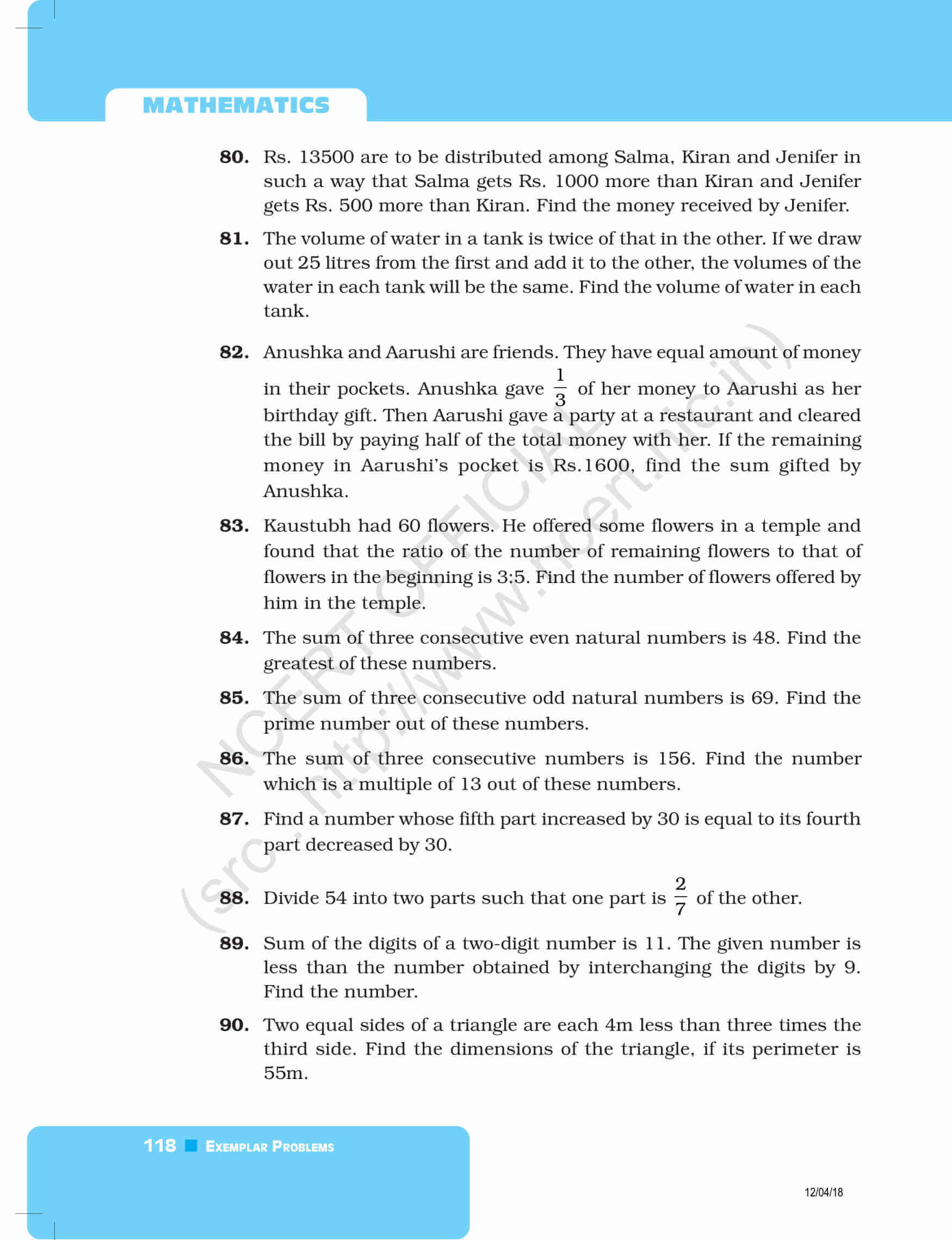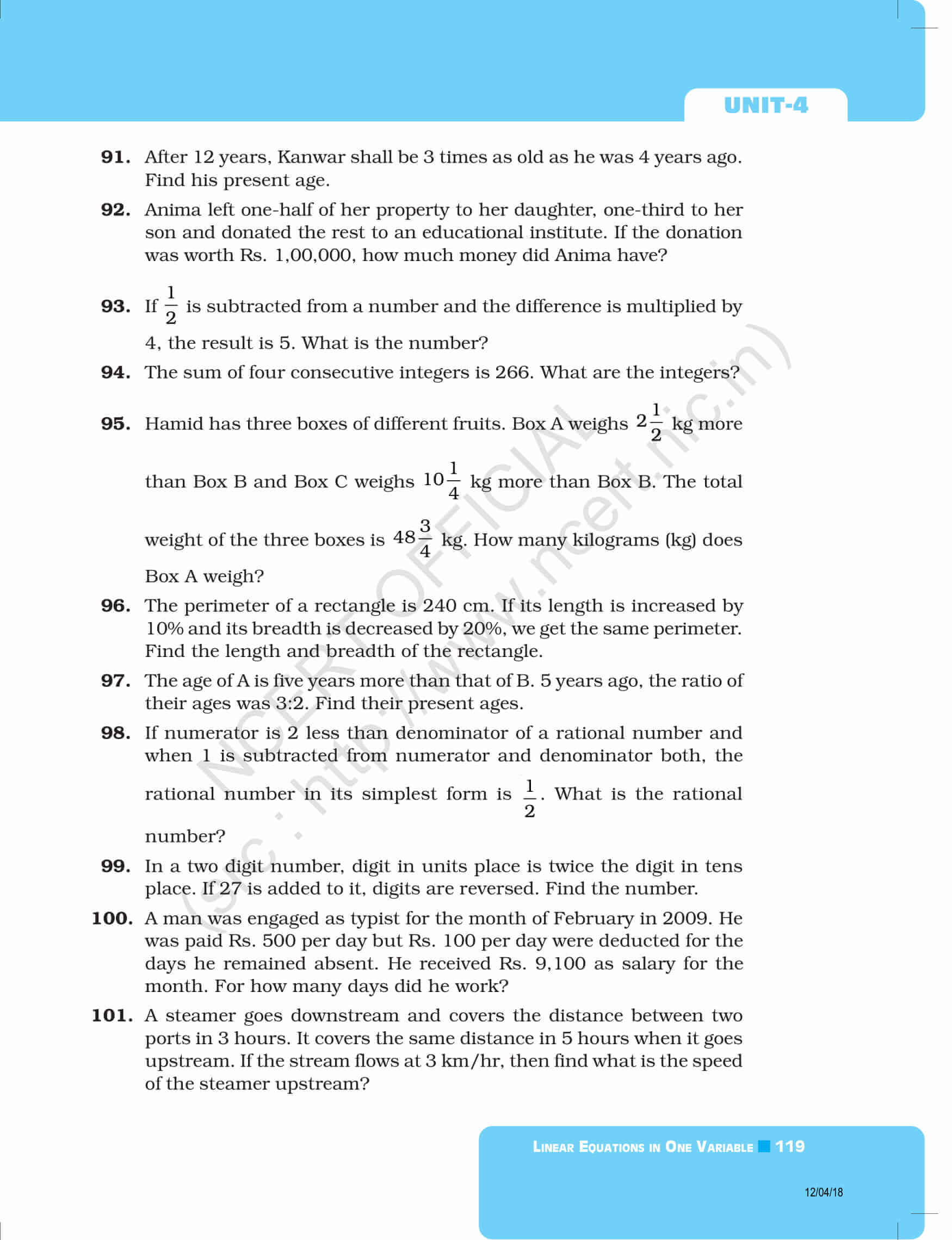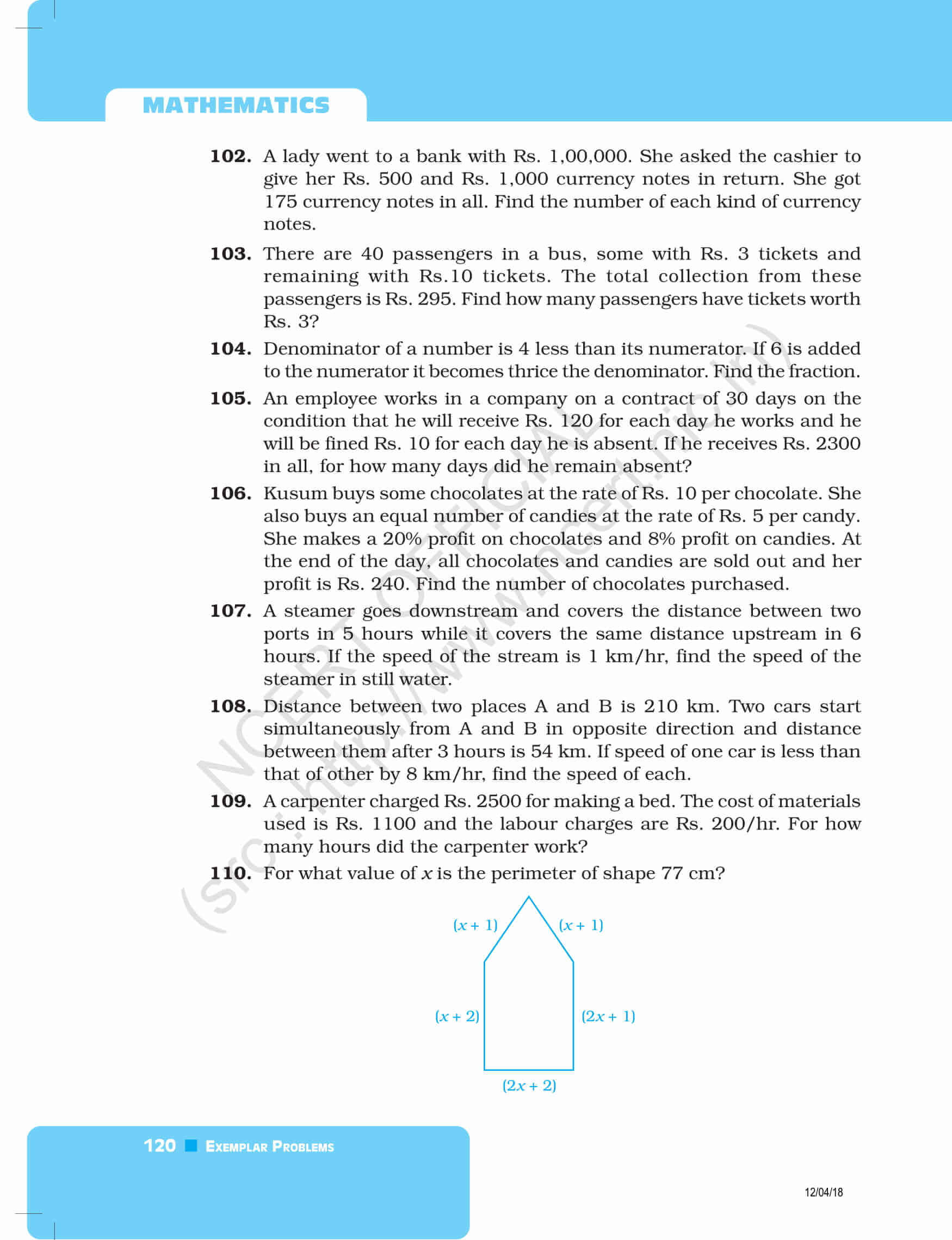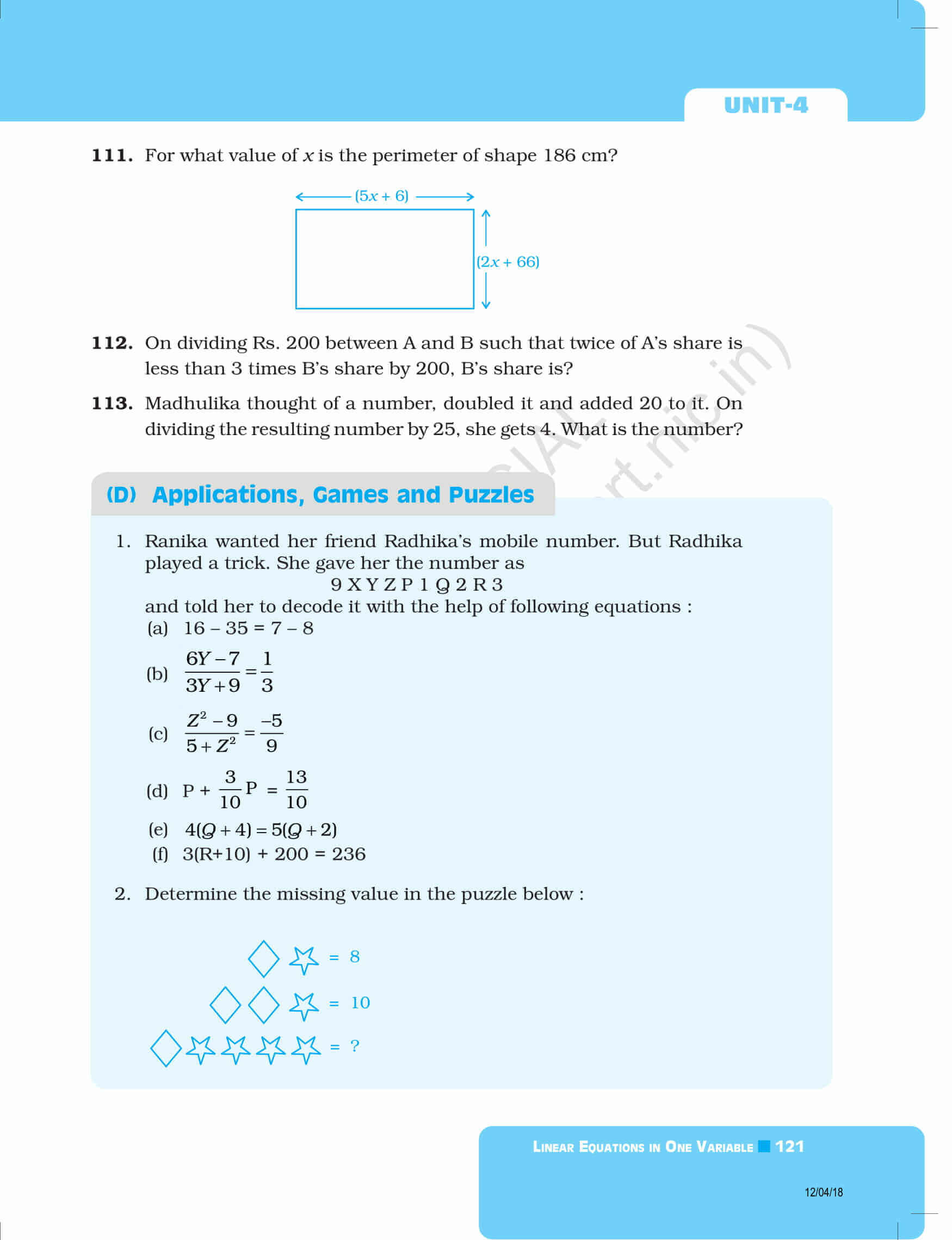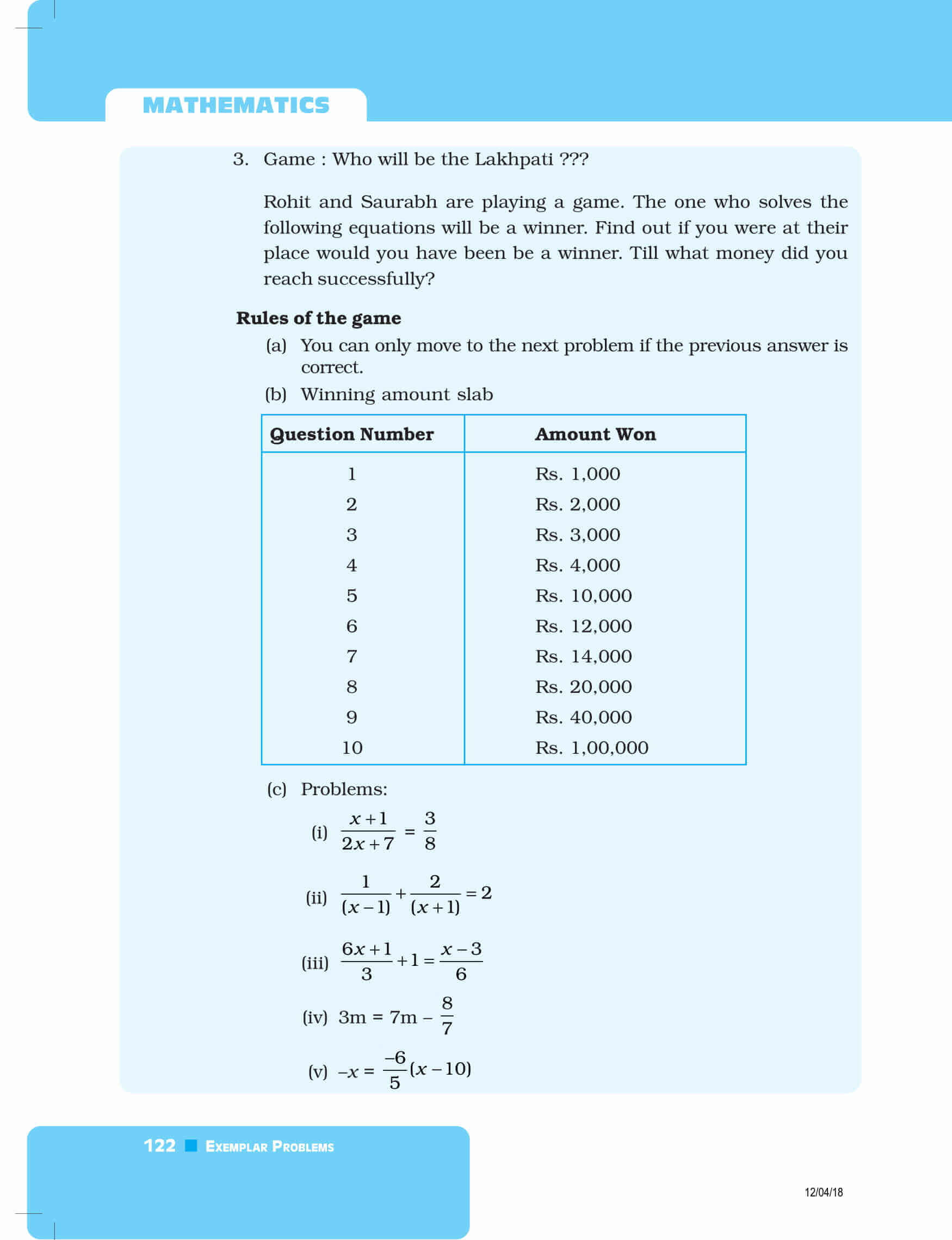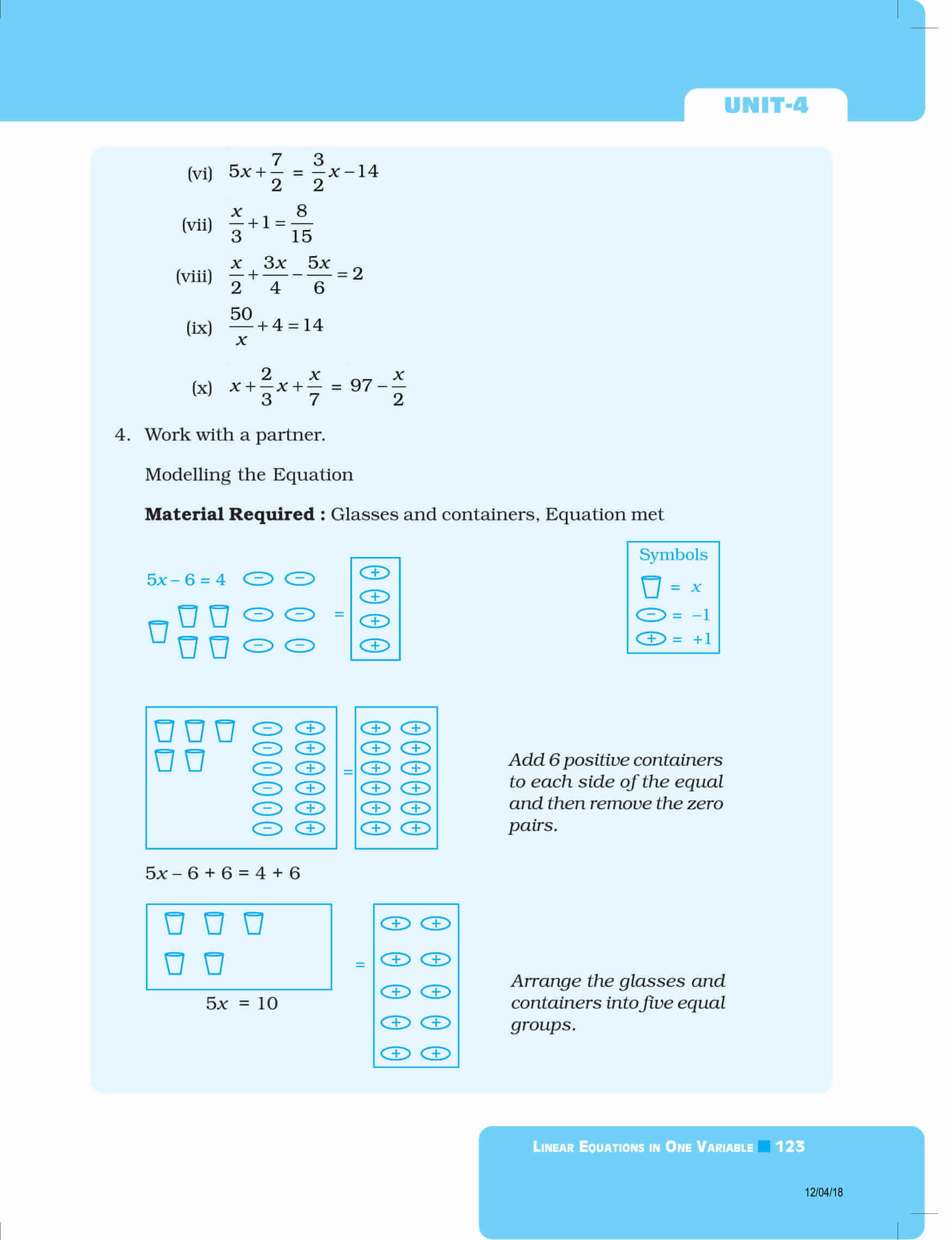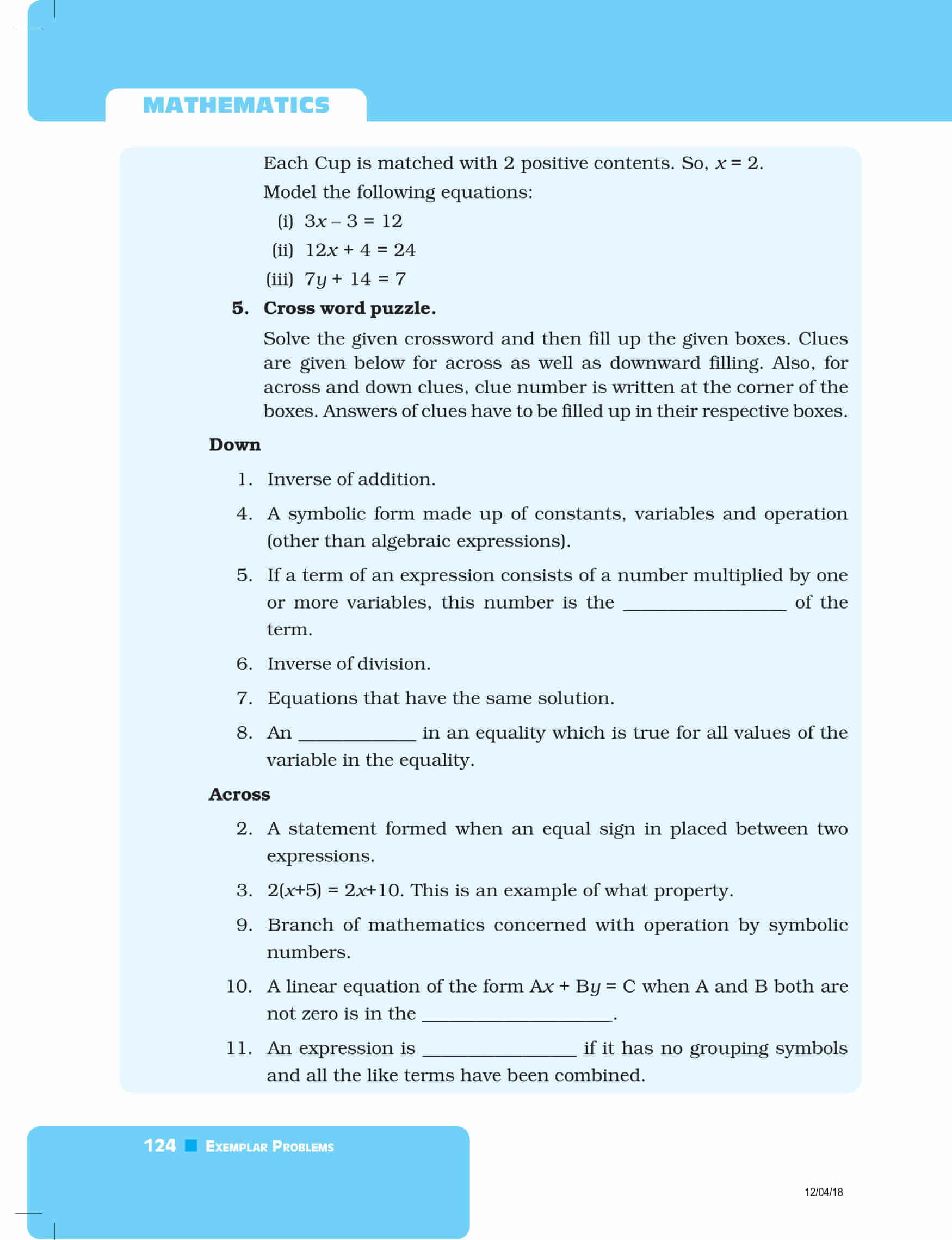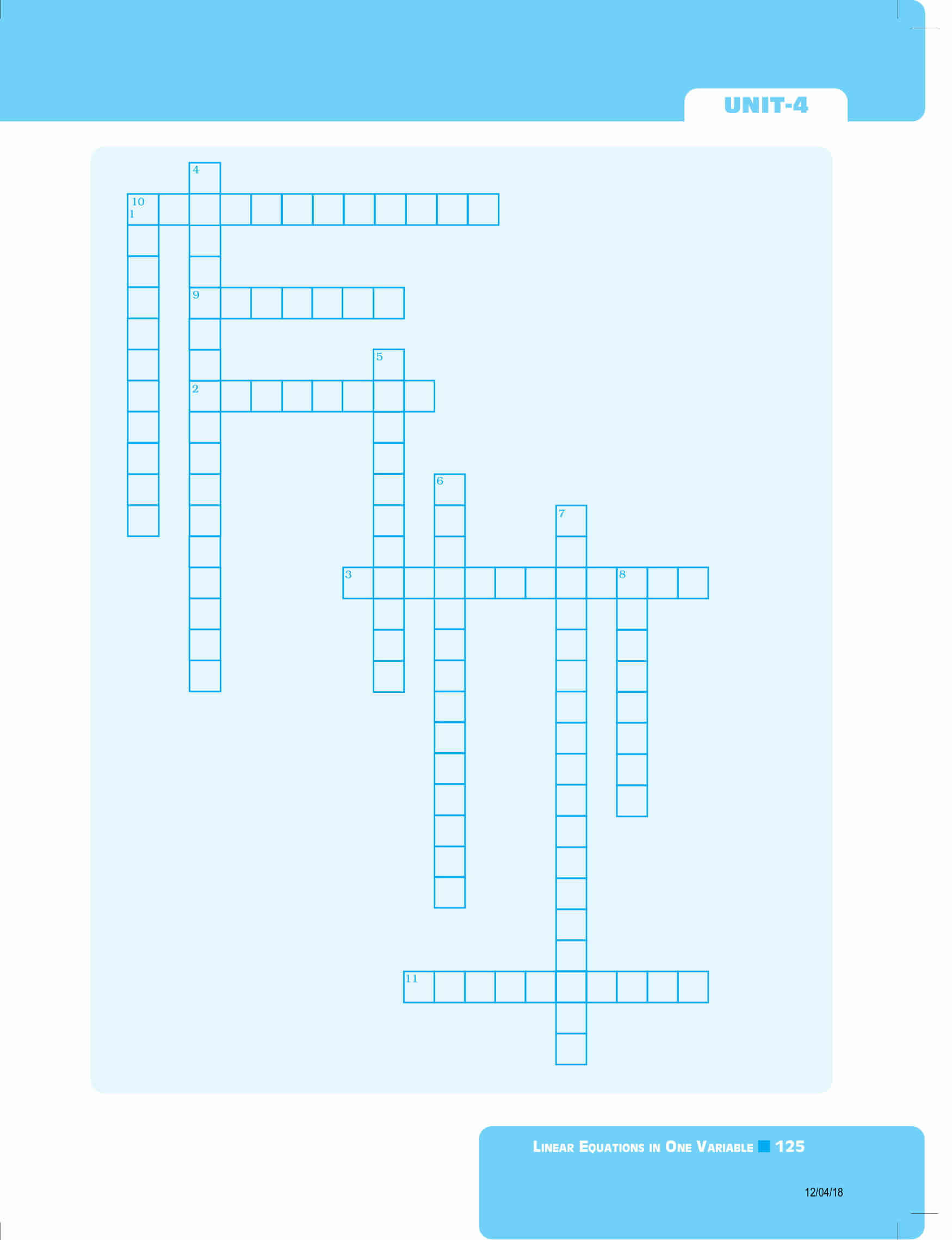Download BYJU’S-The Learning App and get personalized videos, explaining the concepts of linear equations and how to solve them, etc. and experience a new way of learning to understand the concepts easily. Also, get NCERT exemplars for Maths 8th class all the chapters here with us.

Also Visit